Test: Code Generation & Optimization- 2

# Test: Code Generation & Optimization- 2

Test Description

## 15 Questions MCQ Test GATE Computer Science Engineering(CSE) 2023 Mock Test Series | Test: Code Generation & Optimization- 2

Test: Code Generation & Optimization- 2 for Computer Science Engineering (CSE) 2023 is part of GATE Computer Science Engineering(CSE) 2023 Mock Test Series preparation. The Test: Code Generation & Optimization- 2 questions and answers have been prepared according to the Computer Science Engineering (CSE) exam syllabus.The Test: Code Generation & Optimization- 2 MCQs are made for Computer Science Engineering (CSE) 2023 Exam. Find important definitions, questions, notes, meanings, examples, exercises, MCQs and online tests for Test: Code Generation & Optimization- 2 below.
Solutions of Test: Code Generation & Optimization- 2 questions in English are available as part of our GATE Computer Science Engineering(CSE) 2023 Mock Test Series for Computer Science Engineering (CSE) & Test: Code Generation & Optimization- 2 solutions in Hindi for GATE Computer Science Engineering(CSE) 2023 Mock Test Series course. Download more important topics, notes, lectures and mock test series for Computer Science Engineering (CSE) Exam by signing up for free. Attempt Test: Code Generation & Optimization- 2 | 15 questions in 30 minutes | Mock test for Computer Science Engineering (CSE) preparation | Free important questions MCQ to study GATE Computer Science Engineering(CSE) 2023 Mock Test Series for Computer Science Engineering (CSE) Exam | Download free PDF with solutions
 1 Crore+ students have signed up on EduRev. Have you?
Test: Code Generation & Optimization- 2 - Question 1

### In some phase of compiler  Input temp1 := Inttoreal (60) temp2 := id3 * temp1  temp3 := id2 + temp2 id1 := temp3 Output temp 1 := id3 * 60.0 id1 := id2 + temp1 where temp 1 , temp 2 , temp 3 are temporary storage, id1, id2, Id3 are identifier into real is converting int 60 to real number. The above phase is

Detailed Solution for Test: Code Generation & Optimization- 2 - Question 1

Input:
temp1: = inttoreal(60)
temp2: = id3*temp1
temp3 : = id2 + temp2
id1 : = temp3

Output:
temp1 : = id3*60.0
id1 : id2 + temp1

The above phase is code optimization, because it is optimizing the intermediate code using only storages as temp 1 and id1.

Test: Code Generation & Optimization- 2 - Question 2

### The DAG shown here represents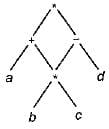Detailed Solution for Test: Code Generation & Optimization- 2 - Question 2

The above DAG represents
(a + b * C ) * { b * C - d )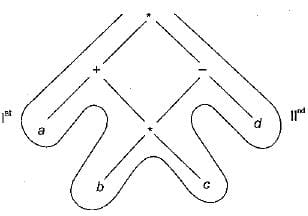Test: Code Generation & Optimization- 2 - Question 3

### Any Directed Acyclic Graph must have

Detailed Solution for Test: Code Generation & Optimization- 2 - Question 3

Simplest DAG: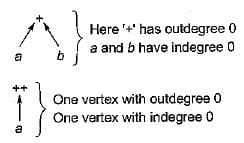Test: Code Generation & Optimization- 2 - Question 4

The DAG shown here represents: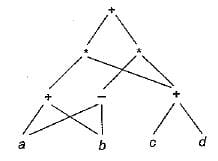Detailed Solution for Test: Code Generation & Optimization- 2 - Question 4

Simplifying the DAG: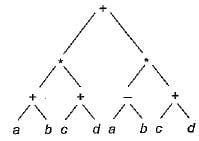Thus result: (a + b) * (c + cf) + (a - b) * (c + d)

Test: Code Generation & Optimization- 2 - Question 5

Consider the DAG with V = {1, 2, 3, 4, 5, 6}, shown below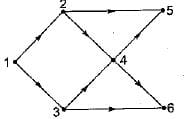Which of the following is NOT a topological ordering

Test: Code Generation & Optimization- 2 - Question 6

A directed acyclic graph represents one form of intermediate representation. The number of non terminals nodes in DAG of a = (b + c)*(b + c) expression is

Detailed Solution for Test: Code Generation & Optimization- 2 - Question 6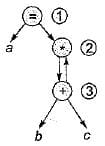Since indegree of node 3 is 1. So cannot start topological ordering with node 3 only start node will be 1.

Test: Code Generation & Optimization- 2 - Question 7

A pictorial representation of the value computed by each statement in the basic block is

Detailed Solution for Test: Code Generation & Optimization- 2 - Question 7

A pictorial representation of the value computed by. each statement in the basic block is control flow graph.

Test: Code Generation & Optimization- 2 - Question 8

DAG representation of a basic block allows

Detailed Solution for Test: Code Generation & Optimization- 2 - Question 8

DAG representation of a basic block allows automatic detection of local common sub expression.

Test: Code Generation & Optimization- 2 - Question 9

The intermediate code generated for the following syntax tree is: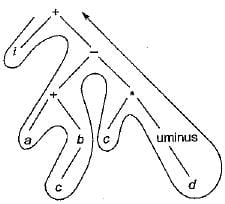Detailed Solution for Test: Code Generation & Optimization- 2 - Question 9

The intermediate code generated is iacb + cd uminus * - +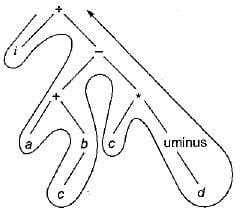Test: Code Generation & Optimization- 2 - Question 10

The triple representation for following representation is: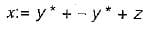Test: Code Generation & Optimization- 2 - Question 11

Generation of intermediate code based on an abstract machine model is useful in compilers because

Detailed Solution for Test: Code Generation & Optimization- 2 - Question 11

Intermediate code enhance the portability of the front end of the compiler.

Test: Code Generation & Optimization- 2 - Question 12

Three address code’ technique for intermediate code generation shows that each statement usually contains three addresses. Three addresses are as follows:

Detailed Solution for Test: Code Generation & Optimization- 2 - Question 12

Three address code formate is two addresses for operands and one for operator.

Test: Code Generation & Optimization- 2 - Question 13

Consider the following arithmetic infix expression Q.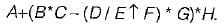Transform 'Q' into its equivalent postfix expression.

Detailed Solution for Test: Code Generation & Optimization- 2 - Question 13

Given expression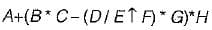Equivalent postfix expression;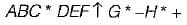Test: Code Generation & Optimization- 2 - Question 14

Which of the following is not a type of three address statements?

Test: Code Generation & Optimization- 2 - Question 15

Consider the following statements:
S1: Three address code is a linearized representation of a syntax tree.
S2: The syntax tree not depicts the natural hierarchical structure of source program

Which of the above statements is/are true

## GATE Computer Science Engineering(CSE) 2023 Mock Test Series

149 docs|215 tests
 Use Code STAYHOME200 and get INR 200 additional OFF Use Coupon Code
Information about Test: Code Generation & Optimization- 2 Page
In this test you can find the Exam questions for Test: Code Generation & Optimization- 2 solved & explained in the simplest way possible. Besides giving Questions and answers for Test: Code Generation & Optimization- 2, EduRev gives you an ample number of Online tests for practice

## GATE Computer Science Engineering(CSE) 2023 Mock Test Series

149 docs|215 tests